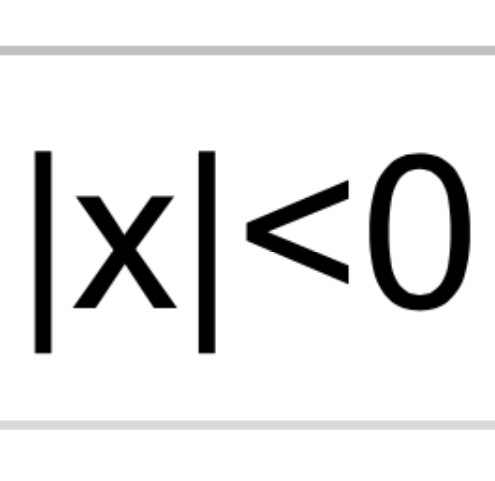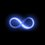# |x|<0. Find x. DNE not accepted.Have fun!

# NOTE:

The problem says "absolute value of x is less than zero". This is NOT a matrix.

# EDIT:

To all of you saying "DNE", you are wrong (as the title says). Here are the hints to guide you out: 1. Do not try to solve this problem using traditional mathematics. 2. Think outside of the realm that lies outside the box (i.e outside of outside the box). 3. The solution is yet as close to infinity as it is far away from it. For each of the seven days that there is no right solution, I'll put up an additional hint.

# HINTS:

Removed.6 years, 5 months ago

This discussion board is a place to discuss our Daily Challenges and the math and science related to those challenges. Explanations are more than just a solution — they should explain the steps and thinking strategies that you used to obtain the solution. Comments should further the discussion of math and science.

When posting on Brilliant:

• Use the emojis to react to an explanation, whether you're congratulating a job well done , or just really confused .
• Ask specific questions about the challenge or the steps in somebody's explanation. Well-posed questions can add a lot to the discussion, but posting "I don't understand!" doesn't help anyone.
• Try to contribute something new to the discussion, whether it is an extension, generalization or other idea related to the challenge.

MarkdownAppears as
*italics* or _italics_ italics
**bold** or __bold__ bold
- bulleted- list
• bulleted
• list
1. numbered2. list
1. numbered
2. list
Note: you must add a full line of space before and after lists for them to show up correctly
paragraph 1paragraph 2

paragraph 1

paragraph 2

[example link](https://brilliant.org)example link
> This is a quote
This is a quote
    # I indented these lines
# 4 spaces, and now they show
# up as a code block.

print "hello world"
# I indented these lines
# 4 spaces, and now they show
# up as a code block.

print "hello world"
MathAppears as
Remember to wrap math in $$ ... $$ or $ ... $ to ensure proper formatting.
2 \times 3 $2 \times 3$
2^{34} $2^{34}$
a_{i-1} $a_{i-1}$
\frac{2}{3} $\frac{2}{3}$
\sqrt{2} $\sqrt{2}$
\sum_{i=1}^3 $\sum_{i=1}^3$
\sin \theta $\sin \theta$
\boxed{123} $\boxed{123}$

Sort by:

it's been too long and as nobody has solved this i highly doubt it has an established answer among the maths community, i would like to hear what the solution to this is, would be very educational i presume

- 4 years, 8 months ago

O-oh...? Did you not get my super-secret 12-hour response a while ago? I explained the concept and told you I will delete it in twelve hours. Whoops. Buut... now this thread is too popular for me to post it again. So, I'll direct you to the guy who found a different type of answer. Erm... an attempt at an answer, rather.

Behold, Mr. Mathopedia: @Michael Mendrin

- 4 years, 8 months ago

clearly its a ruse and there is no answer lol, nice try tho i guess, you at least stimulated some fun discussion with this thread

- 4 years, 8 months ago

Yeah you're right there is no "answer" - it's rather a philosophical thing. But... it's not something that doesn't make sense at all either. One day I shall reveal the... thing.

Till then, keep doing the lame-old math ;)

- 4 years, 8 months ago

John, it might be of interest to you that no less than Stephen Hawkins has co-authored a paper, first published in 2002, titled, "Living with Ghosts", where he addresses the problem of "ghost states" in mathematical quantum field theory, "which have been though to be a fatal flaw in any quantum field theory". What is such a "ghost state"? Why, states that "spuriously" arise mathematically that have NEGATIVE NORMS. Just like your mystery expression you've posted as the basis of this note. Such things sprout like weeds, much to the annoyance of such professional theoretical physicists as the illustrious Stephen Hawkings.

This link may or may not work

Living with Ghosts

The link is from Stephen's own website

- 4 years, 8 months ago

Oh boy here we go with chicken [scratch] math. I'll bookmark this for until when I graduate college LOL

But GREAT TO HEAR HAH!! ACTUALLY YOU WOULD'VE GIVEN UP IF I TOLD YOU THAT $x$ ALSO HAS THE FOLLOWING PROPERTIES:

$|x|<0$

$x>\infty$

$x<-\infty$

$x+a=x, a\neq 0$

TELL ME, IS STEPHEN TOUGH ENOUGH FOR THIS?? xD

- 4 years, 8 months ago

Oh, NaN, NaN, NaN, NaN! I think you're making up stories now.

also check out this, see "Not a Number"

- 4 years, 8 months ago

Don't get me wrong, but the answer is DEFINITELY not a number.

- 4 years, 8 months ago

NaN is a special binary string used in computers that signify "end of order", and thus can either be greater than infinity or less than negative infinity. And if anything should be added to it, the result is unchanged. It would have all the properties you've described for $x$.

- 4 years, 8 months ago

END OF ORDER??!? OOOOMG THAT IS SO CLOSE!!! Like... like... as close as $-2$ is to $2$ ... But at the same time very incomplete. Hmm...........

Y'know... I'm supposed to write ten books about myself (yeah you heard that right), and in Book X I reveal the answer. Buuut... you may be teh first to hear it after all... wooo...

- 4 years, 8 months ago

I think I'll live long enough to see Book X. Maybe I should stop climbing rock?

- 4 years, 8 months ago

Stop climbing?? "Um yeah that's great I'll live long enough for it. Just let me stop living a healthy lifestyle meanwhile." LOL.

(P.S. > you... may not have to worry about "dying" at all... y'know... 2045... wOooOooO....)

(P.S.${}^2$ Avatars A, B, and C coming way before that!)

- 4 years, 8 months ago

After decades of effort, physicists using the Large Hadron Collider at CERN have found the $x$ in that expression. It is inside between the vertical bars.

- 4 years, 8 months ago

Here's a solution set where $|x|<0$ for any $x\in\mathbb{O}$ (Octonion):



$\Huge \{\}$

- 5 years, 1 month ago

Octonions don't work. Octonions is a "normed algebra", i.e., has non-negative norms. Nice try, though. Check out sedenions, though, which don't have this property.

- 4 years, 8 months ago

Hm that looks quite promising! Though I have no idea what Octonions are, despite having read about them briefly though your link, your little "solution" is almost on-point with mine.

However, I'd like to admit that it is merely a clever hypothesis rather than an accepted theorem or solution, but I like to keep it objective to not scare anyone away. If you could perhaps explain a little more what you mean by ${}$, we could be getting on something greater than even I could envision.

- 5 years, 1 month ago

its been so long and i don't think anybody is close to answering this, could you just explain what it is?

- 5 years, 6 months ago

If something is less than $0,$ then it is also less than every number greater than $0.$ Thus, if $|x|<0,$ then $|x|<3.$ This shows that the person loves the absolute value function because <3 is a heart. All we need to do is prove that someone loves the absolute value function. I love the absolute value function, so it is indeed possible. $\mathbb{Q.E.D.}$

- 5 years, 11 months ago

Since I've been absent for 2 weeks, you get 2 hints.

- 6 years, 4 months ago

for me this is peculiar since the other day i had a thought about a number with a smaller magnitude than 0 and thought i'd stumbled upon some new realm of mathematics lol. this is my best attempt at it, though im pretty sure its fraught with logical errors i thought i'd give it a try xD |x|<0 ---> |x|=-k (k is a positive real number) ---> e^|x|=e^-k ---> e^(|x|+k)=1 ---> |x|+k=(2πn)i ---> |x|=-k+(2πn)i ---> x=inverseabs(-k+(2πn)i) ---> |A+Bi|=-k+(2πn)i =sqrt(A^2+B^2) ---> A^2+B^2=k^2-4πkni-(4(π^2)(n^2)) ---> B=sqrt(k^2-4πkni-4(nπ)^2-A^2) --->

x=A+sqrt(k^2-4πkni-4(nπ)^2-A^2)i

there is no way thats right lol

- 6 years, 4 months ago

Here's an overview, you've stumbled upon a problem with identities on complex exponentiation and logarithms.

- 6 years, 4 months ago

It's good you've been thinking of it. Try this app: "The Room". Believe me, you won't regret it ;)

- 6 years, 4 months ago

Have you finished THE ROOM2 yet?

- 5 years, 11 months ago

The Room 3 will be its ultimate conclusion!

- 5 years, 6 months ago

ok i will :) thanks

- 6 years, 4 months ago

To all of you saying "DNE", you are wrong (as the title says). Here are the hints to guide you out: 1. Do not try to solve this problem using traditional mathematics. 2. Think outside of the realm that lies outside the box (i.e outside of outside the box). 3. The solution is yet as close to infinity as it is far away from it. For each of the seven days that there is no right solution, I'll put up an additional hint.

- 6 years, 4 months ago

Do not try to solve this problem using traditional mathematics.

Never mind, then. I thought this was an actual problem to solve.

- 6 years, 4 months ago

"An actual problem"? Any problem that can be solved is an actual problem. There's no such thing as "Actual" and "Not-Actual"

- 6 years, 4 months ago

- 6 years, 4 months ago

Nice try, but no.

- 6 years, 4 months ago

It's not $i$ since the norm of it is 1.

What is very much possible is to have $x$ be a matrix with a negative determinant, thus the condition holds. But apart from that, norms are defined to be nonnegative on inner product spaces, so this only happens on very specific cases.

- 6 years, 4 months ago

This is NOT a matrix.

- 6 years, 4 months ago

There is no solution.Or if this is a math joke,$x$ is between the vertical lines.

- 6 years, 5 months ago

Only solution might be when x represents a matrix but in that case it would represent a determinant symbol

- 6 years, 5 months ago

To everyone coming up with complex numbers:

For a complex number of the form $a+bi$, we have that $|a+bi|=\sqrt{a^2+b^2}$.

Therefore we have that $|i|=|0+1*i|=\sqrt{0^2+1^2}=\sqrt(1)=1$

Also, $i^2=-1$, so $|i^2|=|-1|=1$

- 6 years, 5 months ago

The correct answer is i^2. Since i=square root of -1, then i^2 = -1. => imaginary numbers

- 6 years, 5 months ago

$x=i^2$ gives $|x|=|i^2|=|-1|=1\ge 0$

- 6 years, 5 months ago

DNE

- 6 years, 5 months ago

No solution for $x$.

If you want a answer that isn't that, \begin{aligned}\ |&x|<0 \\ &\uparrow \\ \text{Right}&\text{here} \end{aligned}

- 6 years, 5 months ago

Haha, I know what book you got that from. I love that. :D

- 5 years, 11 months ago

Which book is that?

- 5 years, 11 months ago

I don't remember the title, but it's full of stupid responses to test problems, like

Find $x$:

And then the person draws an arrow towards $x$, and other stupid stuff like that. It's hilarious. :D

- 5 years, 11 months ago

F on exams.

- 5 years, 11 months ago

YES! :D

- 5 years, 11 months ago

A on creativity :)

- 5 years, 11 months ago

x = i

- 6 years, 5 months ago

If $x=i$ then $|x|=1>0$ as per the definition of modulus of a complex number.

- 6 years, 4 months ago